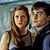# Only People With a GPA Above 3.5 Can Score 100% on This Math Quiz

## Are you up to speed on your algebra? Only the smartest and brightest can score on this intensive math quiz.Created by Carly Wallace
On Aug 28, 2023

Does it matter what your GPA is in high school or University? Some would argue that it does! But then again, there are tons of extremely smart people who have low GPAs. Try and remember your algebra and solve these equations. Let’s find out if your GPA matters in scoring on this difficult math test!

>> Apologies in Advance, But We Doubt You're Smarter Than an Elephant

1 / 10

Evaluate the expression:
4x -2 when x = 3.

2 / 10

Solve for y:
3y + 9 = 24

3 / 10

Solve the equation for x:
2x - 6 = 10

4 / 10

Solve the inequality:
−3x + 2 < 5

​.

5 / 10

Find the x-intercept(s) of the equation: x= 2x − 6
y= 2x − 6.

6 / 10

Simplify: (3x−7) + (7−3x)

7 / 10

Evaluate the expression: 2x+6
2y+6 when x=−3
y=−3.

8 / 10

Solve for x
x: 4x + 10 = 26

9 / 10

Solve the equation for y:
y: 5y + 7= −3

10 / 10

Solve the inequality: 2x − 5 > 8

10
Questions left

### What STEM subject would you excel in?

Calculating results
These are 10 of the World CRAZIEST Ice Cream Flavors
Created by Tal Garner
On Nov 18, 2021